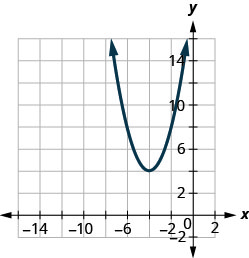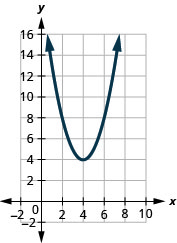$$\newcommand{\id}{\mathrm{id}}$$ $$\newcommand{\Span}{\mathrm{span}}$$ $$\newcommand{\kernel}{\mathrm{null}\,}$$ $$\newcommand{\range}{\mathrm{range}\,}$$ $$\newcommand{\RealPart}{\mathrm{Re}}$$ $$\newcommand{\ImaginaryPart}{\mathrm{Im}}$$ $$\newcommand{\Argument}{\mathrm{Arg}}$$ $$\newcommand{\norm}{\| #1 \|}$$ $$\newcommand{\inner}{\langle #1, #2 \rangle}$$ $$\newcommand{\Span}{\mathrm{span}}$$

# 9.8E: Exercises

•• OpenStax
• OpenStax
$$\newcommand{\vecs}{\overset { \rightharpoonup} {\mathbf{#1}} }$$ $$\newcommand{\vecd}{\overset{-\!-\!\rightharpoonup}{\vphantom{a}\smash {#1}}}$$$$\newcommand{\id}{\mathrm{id}}$$ $$\newcommand{\Span}{\mathrm{span}}$$ $$\newcommand{\kernel}{\mathrm{null}\,}$$ $$\newcommand{\range}{\mathrm{range}\,}$$ $$\newcommand{\RealPart}{\mathrm{Re}}$$ $$\newcommand{\ImaginaryPart}{\mathrm{Im}}$$ $$\newcommand{\Argument}{\mathrm{Arg}}$$ $$\newcommand{\norm}{\| #1 \|}$$ $$\newcommand{\inner}{\langle #1, #2 \rangle}$$ $$\newcommand{\Span}{\mathrm{span}}$$ $$\newcommand{\id}{\mathrm{id}}$$ $$\newcommand{\Span}{\mathrm{span}}$$ $$\newcommand{\kernel}{\mathrm{null}\,}$$ $$\newcommand{\range}{\mathrm{range}\,}$$ $$\newcommand{\RealPart}{\mathrm{Re}}$$ $$\newcommand{\ImaginaryPart}{\mathrm{Im}}$$ $$\newcommand{\Argument}{\mathrm{Arg}}$$ $$\newcommand{\norm}{\| #1 \|}$$ $$\newcommand{\inner}{\langle #1, #2 \rangle}$$ $$\newcommand{\Span}{\mathrm{span}}$$

### Practice Makes Perfect

##### Exercise $$\PageIndex{23}$$ Graph Quadratic Functions of the Form $$f(x)=x^{2}=k$$

In the following exercises,

1. Graph the quadratic functions on the same rectangular coordinate system
2. Describe what effect adding a constant, $$k$$, to the function has on the basic parabola.
1. $$f(x)=x^{2}, g(x)=x^{2}+4, \text { and } h(x)=x^{2}-4$$
2. $$f(x)=x^{2}, g(x)=x^{2}+7, \text { and } h(x)=x^{2}-7$$

1.

1.Figure 9.7.71
2. The graph of $$g(x)=x^{2}+4$$ is the same as the graph of $$f(x)=x^{2}$$ but shifted up $$4$$ units. The graph of $$h(x)=x^{2}-4$$ is the same as the graph of $$f(x)=x^{2}$$ but shift down $$4$$ units.
##### Exercise $$\PageIndex{24}$$ Graph Quadratic Functions of the Form $$f(x)=x^{2}=k$$

In the following exercises, graph each function using a vertical shift.

1. $$f(x)=x^{2}+3$$
2. $$f(x)=x^{2}-7$$
3. $$g(x)=x^{2}+2$$
4. $$g(x)=x^{2}+5$$
5. $$h(x)=x^{2}-4$$
6. $$h(x)=x^{2}-5$$

1.

3.

5.

##### Exercise $$\PageIndex{25}$$ Graph Quadratic Functions of the Form $$f(x)=(x-h)^{2}$$

In the following exercises,

1. Graph the quadratic functions on the same rectangular coordinate system
2. Describe what effect adding a constant, $$h$$, inside the parentheses has
1. $$f(x)=x^{2}, g(x)=(x-3)^{2}, \text { and } h(x)=(x+3)^{2}$$
2. $$f(x)=x^{2}, g(x)=(x+4)^{2}, \text { and } h(x)=(x-4)^{2}$$

1.

1.Figure 9.7.75
2. The graph of $$g(x)=(x−3)^{2}$$ is the same as the graph of $$f(x)=x^{2}$$ but shifted right $$3$$ units. The graph of $$h(x)=(x+3)^{2}$$ is the same as the graph of $$f(x)=x^{2}$$ but shifted left $$3$$ units.
##### Exercise $$\PageIndex{26}$$ Graph Quadratic Functions of the Form $$f(x)=(x-h)^{2}$$

In the following exercises, graph each function using a horizontal shift.

1. $$f(x)=(x-2)^{2}$$
2. $$f(x)=(x-1)^{2}$$
3. $$f(x)=(x+5)^{2}$$
4. $$f(x)=(x+3)^{2}$$
5. $$f(x)=(x-5)^{2}$$
6. $$f(x)=(x+2)^{2}$$

1.

3.

5.

##### Exercise $$\PageIndex{27}$$ Graph Quadratic Functions of the Form $$f(x)=(x-h)^{2}$$

In the following exercises, graph each function using transformations.

1. $$f(x)=(x+2)^{2}+1$$
2. $$f(x)=(x+4)^{2}+2$$
3. $$f(x)=(x-1)^{2}+5$$
4. $$f(x)=(x-3)^{2}+4$$
5. $$f(x)=(x+3)^{2}-1$$
6. $$f(x)=(x+5)^{2}-2$$
7. $$f(x)=(x-4)^{2}-3$$
8. $$f(x)=(x-6)^{2}-2$$

1.

3.

5.

7.

##### Exercise $$\PageIndex{28}$$ Graph Quadratic Functions of the Form $$f(x)=ax^{2}$$

In the following exercises, graph each function.

1. $$f(x)=-2 x^{2}$$
2. $$f(x)=4 x^{2}$$
3. $$f(x)=-4 x^{2}$$
4. $$f(x)=-x^{2}$$
5. $$f(x)=\frac{1}{2} x^{2}$$
6. $$f(x)=\frac{1}{3} x^{2}$$
7. $$f(x)=\frac{1}{4} x^{2}$$
8. $$f(x)=-\frac{1}{2} x^{2}$$

1.

3.

5.

7.

##### Exercise $$\PageIndex{29}$$ Graph Quadratic Functions Using Transformations

In the following exercises, rewrite each function in the $$f(x)=a(x−h)^{2}+k$$ form by completing the square.

1. $$f(x)=-3 x^{2}-12 x-5$$
2. $$f(x)=2 x^{2}-12 x+7$$
3. $$f(x)=3 x^{2}+6 x-1$$
4. $$f(x)=-4 x^{2}-16 x-9$$

1. $$f(x)=-3(x+2)^{2}+7$$

3. $$f(x)=3(x+1)^{2}-4$$

##### Exercise $$\PageIndex{30}$$ Graph Quadratic Functions Using Transformations

In the following exercises,

1. Rewrite each function in $$f(x)=a(x−h)^{2}+k$$ form
2. Graph it by using transformations
1. $$f(x)=x^{2}+6 x+5$$
2. $$(x)=x^{2}+4 x-12$$
3. $$f(x)=x^{2}+4 x-12$$
4. $$f(x)=x^{2}-6 x+8$$
5. $$f(x)=x^{2}-6 x+15$$
6. $$f(x)=x^{2}+8 x+10$$
7. $$f(x)=-x^{2}+8 x-16$$
8. $$f(x)=-x^{2}+2 x-7$$
9. $$f(x)=-x^{2}-4 x+2$$
10. $$f(x)=-x^{2}+4 x-5$$
11. $$f(x)=5 x^{2}-10 x+8$$
12. $$f(x)=3 x^{2}+18 x+20$$
13. $$f(x)=2 x^{2}-4 x+1$$
14. $$f(x)=3 x^{2}-6 x-1$$
15. $$f(x)=-2 x^{2}+8 x-10$$
16. $$f(x)=-3 x^{2}+6 x+1$$

1.

1. f(x)=(x+3)^{2}-4

3.

1. $$f(x)=(x+2)^{2}-1$$

5.

1. $$f(x)=(x-3)^{2}+6$$

7.

1. $$f(x)=-(x-4)^{2}+0$$

9.

1. $$f(x)=-(x+2)^{2}+6$$

11.

1. $$f(x)=5(x-1)^{2}+3$$

13.

1. $$f(x)=2(x-1)^{2}-1$$

15.

1. $$f(x)=-2(x-2)^{2}-2$$
##### Exercise $$\PageIndex{31}$$ Graph Quadratic Functions Using Transformations

In the following exercises,

1. Rewrite each function in $$f(x)=a(x−h)^{2}+k$$ form
2. Graph it using properties
1. $$f(x)=2 x^{2}+4 x+6$$
2. $$f(x)=3 x^{2}-12 x+7$$
3. $$f(x)=-x^{2}+2 x-4$$
4. $$f(x)=-2 x^{2}-4 x-5$$

1.

1. $$f(x)=2(x+1)^{2}+4$$

3.

1. $$f(x)=-(x-1)^{2}-3$$
##### Exercise $$\PageIndex{32}$$ Matching

In the following exercises, match the graphs to one of the following functions:

1. $$f(x)=x^{2}+4$$
2. $$f(x)=x^{2}-4$$
3. $$f(x)=(x+4)^{2}$$
4. $$f(x)=(x-4)^{2}$$
5. $$f(x)=(x+4)^{2}-4$$
6. $$f(x)=(x+4)^{2}+4$$
7. $$f(x)=(x-4)^{2}-4$$
8. $$f(x)=(x-4)^{2}+4$$

1.Figure 9.7.97

2.Figure 9.7.98

3.Figure 9.7.99

4.Figure 9.7.100

5.Figure 9.7.101

6.Figure 9.7.102

7.Figure 9.7.103

8.Figure 9.7.104

1. c

3. e

5. d

7. g

##### Exercise $$\PageIndex{33}$$ Find a Quadratic Function from its Graph

In the following exercises, write the quadratic function in $$f(x)=a(x−h)^{2}+k$$ form whose graph is shown.

1.Figure 9.7.105

2.Figure 9.7.106

3.Figure 9.7.107

4.Figure 9.7.108

1. $$f(x)=(x+1)^{2}-5$$

3. $$f(x)=2(x-1)^{2}-3$$

##### Exercise $$\PageIndex{34}$$ Writing Exercise
1. Graph the quadratic function $$f(x)=x^{2}+4x+5$$ first using the properties as we did in the last section and then graph it using transformations. Which method do you prefer? Why?
2. Graph the quadratic function $$f(x)=2x^{2}−4x−3$$ first using the properties as we did in the last section and then graph it using transformations. Which method do you prefer? Why?